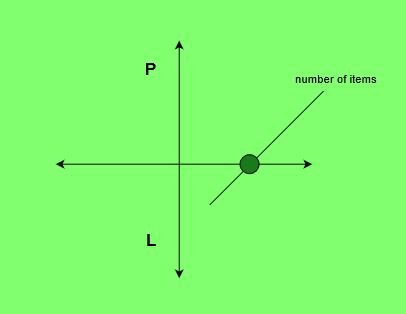# Program to find the Break Even Point

Given the list of monthly expenditureof an organization, selling priceand the overhead maintenanceof each item, the task is to calculate the Break Even Point.
Break Even Point refers to the number of items sold in order to neutralize the total expenditure i.e. Overall, neither profit nor loss.

Examples:

Input: Expenditure = 18000, S = 600, M = 100
Output: 36
We need to sell 36 items to cover expenditure and maintenance overhead

Input: Expenditure = 3550, S = 90, M = 65
Output: 142## Recommended: Please try your approach on {IDE} first, before moving on to the solution.

Approach:

1. Calculate the sum of all the expenditures.
2. Subtract the maintenance (Cost price) from the selling price.
3. Divide the expenditure sum by the above-obtained amount to get the minimum number of items to be sold (Break Even Point).

Below is the implementation of the above approach:

## C++

 // C++ program to find the break-even point.     #include  #include  using namespace std;     // Function to calculate Break Even Point  int breakEvenPoint(int exp, int S, int M)  {      float earn = S - M;         // Calculating number of articles to be sold      int res = ceil(exp / earn);         return res;  }     // Main Function  int main()  {      int exp = 3550, S = 90, M = 65;      cout << breakEvenPoint(exp, S, M);      return 0;  }

## Java

 // Java program to find Break Even Point  import java.io.*;  import java.lang.*;     class GFG  {  // Function to calculate   // Break Even Point  public static int breakEvenPoint(int exp1,                                    int S, int M)  {      double earn = S - M;              double exp = exp1;         // Calculating number of      // articles to be sold       double res = Math.ceil(exp / earn);          int res1 = (int) res;             return res1;  }     // Driver Code  public static void main (String[] args)  {      int exp = 3550, S = 90, M = 65;       System.out.println(breakEvenPoint(exp, S, M));   }  }     // This code is contributed  // by Naman_Garg

## Python 3

 # Python 3 program to find   # Break Even Point  import math     # Function to calculate   # Break Even Point  def breakEvenPoint(exp, S, M):             earn = S - M         # Calculating number of       # articles to be sold       res = math.ceil(exp / earn)                 return res         # Driver Code  if __name__ == "__main__" :                 exp = 3550     S = 90     M = 65            print (int(breakEvenPoint(exp, S, M)))     # This code is contributed  # by Naman_Garg

## C#

 // C# program to find Break Even Point  using System;     class GFG  {  // Function to calculate   // Break Even Point  public static int breakEvenPoint(int exp1,                                   int S, int M)  {      double earn = S - M;              double exp = exp1;         // Calculating number of      // articles to be sold       double res = Math.Ceiling(exp / earn);          int res1 = (int) res;             return res1;  }     // Driver Code  public static void Main ()  {      int exp = 3550, S = 90, M = 65;       Console.WriteLine(breakEvenPoint(exp, S, M));   }  }     // This code is contributed  // by inder_verma..

## PHP

 

Output:

142


My Personal Notes arrow_drop_upTechie Geek

If you like GeeksforGeeks and would like to contribute, you can also write an article using contribute.geeksforgeeks.org or mail your article to contribute@geeksforgeeks.org. See your article appearing on the GeeksforGeeks main page and help other Geeks.

Please Improve this article if you find anything incorrect by clicking on the "Improve Article" button below.

Improved By : Naman_Garg, inderDuMCA